Courses
Courses for Kids
Free study material
Free LIVE classes
More

# Understanding Decimal ComparisonLIVE
Join Vedantu’s FREE Mastercalss

## Introduction

Decimal numbers are quite often seen in our day-to-day life. It is useful for the calculation in our daily life but also by the scientist as it could not have been possible to find the accurate distance between any two bodies like the sun, moon, stars, etc. The main advantages of the decimal number system are easy readability, use by humans, and ease of manipulation.

## What are decimal numbers?

The number that is present between two consecutive numbers then all such numbers are decimal numbers. There are infinite decimal numbers between two consecutive numbers. Is it confusing? Don't worry we are here to explain well and to clear your doubt. Let us first understand what a decimal number is. A decimal number is a fraction whose numerator is expressed in a figure and the denominator is a power of ten. It consists of two parts: one is a whole number, and the other is a fractional part separated by a decimal point. The dot present between the whole number and the fractional part is called the decimal part.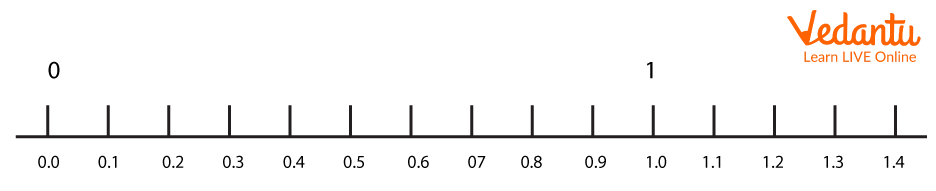Decimal Numbers

You can clearly see there are so many decimal numbers between two consecutive numbers. And we can also clearly see that the first part is a whole number and the second one is a fractional one.

Let us take some examples:

33.45,66.87, 650.98, etc.

## How to compare decimals?

There are some rules to compare decimals:

• While comparing the natural numbers we first compare the total number of digits in both numbers and if they are equal then we compare the digit and the extreme left.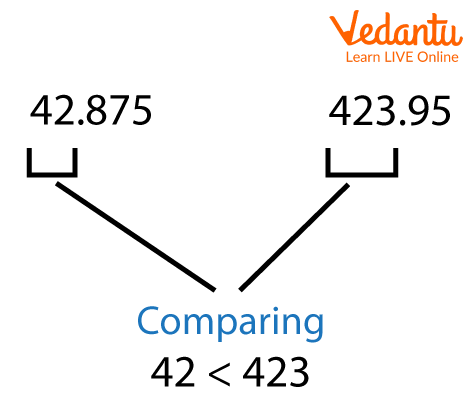Comparing the Natural Numbers

• After that, compare the entire number. Compare them if they are not equal; otherwise, move on to the next stage.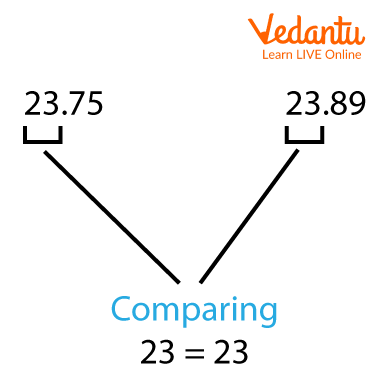Compare the Whole Number

• Compare tenth-place numbers. If the numbers are different then compare them, if not then go to the next step.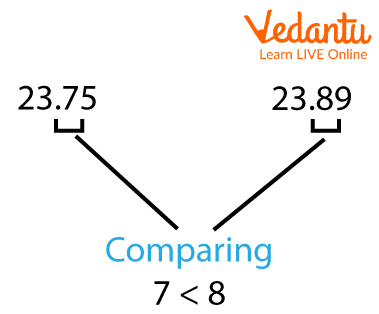Compare Tenth Place Numbers

• Now compare the hundredth number. If the numbers are different then compare them, if not then again go to the next step.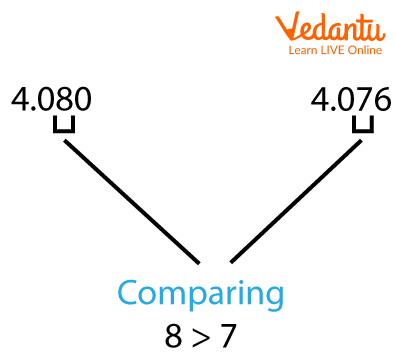Compare the Hundredth Place Number

Etc…..

## Compare decimal number

Steps to the comparison of decimal fractions are given below:

Step 1: First we will compare the whole number.

For example:

• 564> 363.

• 432<876

• \[654 = 654\]

Step 2: When the integer part is the same then compare the tenths part

For example:

• 3.5>3.1

• 7.8<7.9

• 3.8=3.8

Step 3: When the tenths place is the same compare the hundredths place

For example:

• 12.34> 12.31

• 32.76<32.78

• \[56.76 = 56.76\]

In this way, we will compare the number.

For example, 9.8 is greater than 7.7

First, we compare the whole number. If the digits are equal then we will proceed to the next step. But here 9 and 7, that is the whole number is different so from the first step only we could clearly indicate that 9.8 is greater than 7.7.

For example, 4.62 is greater than 4.21

First, we will compare the whole number which is the same in this example. Now we will go to the next step i.e we will compare the tenth place number which is 6 and 2 so we will compare these two, so this state that 4.62 is greater than 4.21

## Solved Examples

1: Which is the smaller number 187.654 or 187.765?

First check the integer number

187=187

Now check the tenth number

6<7

So 187.654< 187.765

2: Find the greater number; 23.54 or 54.76?

First check the integer number

23< 54

So from this, we get to know that 23.54< 54.76

3: Which number is greater 293.82 or 293.62

first check the integer part,

\[293 = 293\]

Then check the tenth place

8> 6

Now check the hundredth place \[2 = 2\]

Therefore, 293.82 > 293.62

## Conclusion

A decimal is a combination of a whole number and a fraction of a whole number. Decimal fraction encourages students to learn about precise quantities. This will help them to understand the weight or distance at an accurate level. By practicing every day with the students it will become quite easy to understand and solve the problem. We can easily compare decimal numbers with daily practice.

Last updated date: 30th Sep 2023
Total views: 70.5k
Views today: 0.70k

## FAQs on Understanding Decimal Comparison

1. What are decimals?

Decimals are numbers that consist of two parts: the first one is the whole number and the number that is after the point is a fractional part. For example,34.56

2. What are the different types of decimals?

The two different types of decimals are:

Terminating decimals and non-terminating decimals

3. How to convert fractions to decimals?

To convert fractions to decimals, divide the numerator by the denominator value.